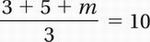# SAT Math Multiple Choice Question 752: Answer and Explanation

### Test Information

Question: 752

2. If the average of 3, 5, and m is 10, what is the value of m?

• A. 2
• B. 6
• C. 12
• D. 22

Explanation:

D

Problem Solving and Data Analysis (central tendency) EASY

The average of the three numbers is 10:Multiply by 3:

3 + 5 + m = 30

Simplify:

8 + m = 30

Subtract 8:

m = 22